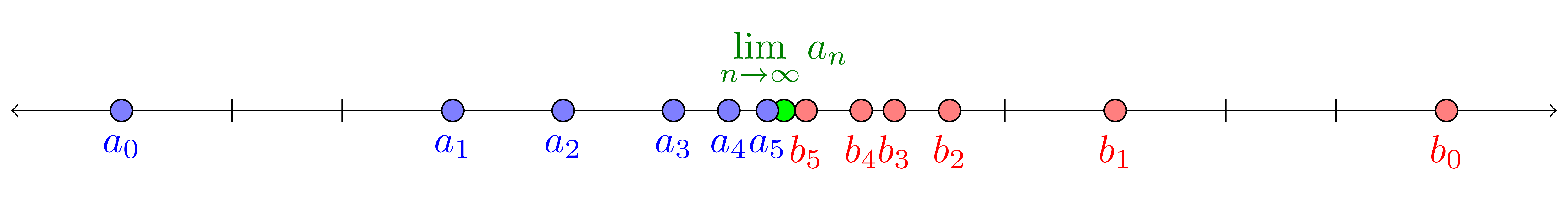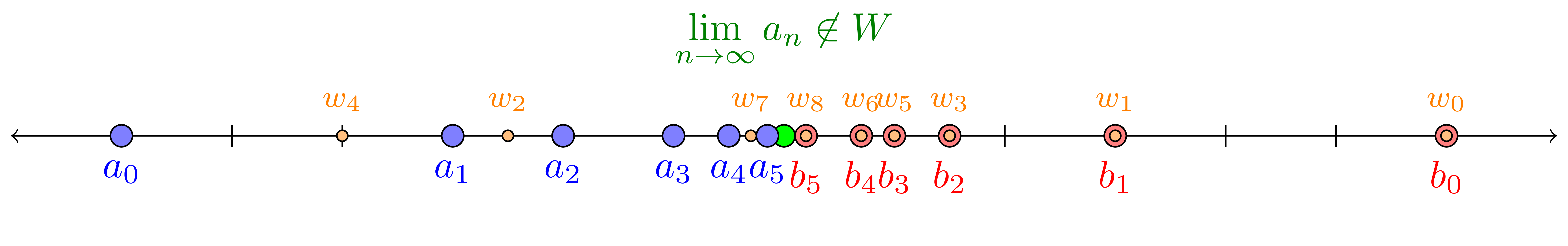# Games Topologists Play

#### 2020 March

Steven Clontz
University of South Alabama

### Abstract

Many topological properties may be characterized in terms of who can guarantee victory (and how much memory they require) in certain two-player, infinite-length games. To illustrate this, we will explore a novel game-theoretic proof of the uncountability of the real numbers, and then discuss the class of "selection games" used throughout general topology.

# An Introductory $$\omega$$-length Game

### The Cantor Game

Let $$W\subseteq\mathbb R\text{.}$$ Then $$CG(W)$$ denotes the following game played by players $$P1$$ and $$P2\text{.}$$

During round $$0\text{,}$$ $$P1$$ chooses some real number $$a_0\text{,}$$ followed by $$P2$$ choosing $$b_0$$ such that $$a_0\lt b_0\text{.}$$

During round $$n+1\text{,}$$ $$P1$$ chooses $$a_{n+1}\in(a_n,b_n)\text{,}$$ followed by $$P2$$ choosing $$b_{n+1}\in(a_{n+1},b_n)\text{.}$$

After $$\omega=\{0,1,2,\dots\}$$-many rounds, $$P1$$ wins this game in the case that $$\displaystyle\lim_{n\to\infty}a_n\in W\text{.}$$(This game seems to be introduced by Grossman and Turett in the problems section of Mathematics Magazine Vol. 71, with some further analysis done by Matt Baker in a later volume.)

### Strategies

It'd take some time to complete this kind of $$\omega$$-length game.

However, each player in such a game can define a strategy $$\sigma:M^{\lt\omega}\to M$$ that designates their moves in response to their opponent's actions...

\begin{equation*} \underbrace{\sigma}_{\text{player strategy}} : \underbrace{M^{\lt\omega}}_{\text{finite sequence of opponent's moves}} \to \underbrace{M}_{\text{player's next move}} \end{equation*}

Typically, we want to prove something about the existence of winning strategies that defeat any counter-strategy chosen by the opponent.

If player $$P$$ has a winning strategy for game $$G\text{,}$$ we write $$P\win G\text{.}$$

### Proposition:

$$P1\win CG(\mathbb R)\text{.}$$

### Theorem:

If $$W$$ is countable, then $$P2\win CG(W)\text{.}$$

### Corollary:

$$\mathbb R$$ is uncountable.

### Theorem:

If $$W$$ is countable, then $$P2\win CG(W)\text{.}$$

Let $$W=\{w_n:n\in\omega\}\text{.}$$ Then if any element of $$W$$ is legal to play during $$P2$$'s turn, their strategy is to play $$w_n\text{,}$$ where $$n$$ is the minimal ordinal such that $$w_n$$ is legal to play. Otherwise, $$P2$$ chooses arbitrarily.At the end of the game, each element $$w_N\in W$$ was either played before round $$N\text{,}$$ or was illegal to be played in round $$N\text{.}$$ Either way, $$w_N\not=\lim_{n\to\infty} a_n\text{,}$$ so $$P2$$'s strategy is winning.

# Selection Games

### Definition

Given two sets $$\mc A,\mc B\text{,}$$ the selection game $$G_1(\mc A,\mc B)$$ is played as follows:$$P2$$ wins this game provided $$\{b_n:n\lt\omega\}\in\mc B\text{.}$$

$$G_{fin}(\mc A,\mc B)$$ is similar, except $$P2$$ can make finitely-many selections each round.

### Topological Examples

Let $$X$$ be some topological space with a particular point $$x\text{.}$$

• $$G_*(\mc O,\mc O)\text{:}$$ P1 chooses open covers of $$X\text{,}$$ P2 tries to build their own open cover.
• $$G_*(\mc D,\Gamma_x)\text{:}$$ P1 chooses dense subsets of $$X\text{,}$$ P2 tries to build a sequence converging to $$x\text{.}$$

### Limited Information

A strategy that can ignore all but the most recent move of the opponent (but might use the current round #) is said to be Markov.

A strategy that can ignore all moves of the opponent (but probably uses the current round #) is said to be predetermined.

For $$X=\mathbb R$$ with the usual topology:

• $$P1\prewin G_1(\mc O,\mc O)$$ but $$P2\markwin G_{fin}(\mc O,\mc O)\text{.}$$
• $$P2\markwin G_1(\mc D,\Gamma_0)$$ (so $$P2\markwin G_{fin}(\mc D,\Gamma_0)$$ also).

### Spectrum of propertiesNotes:

• For second-countable spaces, $$P2\markwin G_*(\mc O,\mc O)\Leftrightarrow P2\win G_*(\mc O,\mc O)\text{.}$$
• Using the Continuum Hypothesis and the Axiom of Choice, one may construct Lusin sets $$X\subseteq\mathbb R\text{.}$$ Any Lusin set satisfies both $$P1\not\win G_*(\mc O,\mc O)$$ and $$P2\not\win G_*(\mc O,\mc O)\text{.}$$
• While $$P1\win G_*(\mc O,\mc O)\Leftrightarrow P1\prewin G_*(\mc O,\mc O)$$ (Galvin 1979, Pawlikowski 1994), F. Jordan (2020) found a space where $$P1\win G_1(\mc K,\mc O)$$ but $$P1\not\prewin G_1(\mc K,\mc O)\text{.}$$

# Dual Selection Games

### The Point-Open Game

Let $$\mc O$$ be the set of open covers, and $$\mc P$$ be the set of point-bases. Let $$\neg$$ denote the complement of a set.

Then the point-open game $$G_1(\mc P,\neg\mc O)$$ essentially has $$P1$$ choosing points, and $$P2$$ selecting a neighborhood for each point. Then $$P1$$ wins if $$P2$$'s sets form a cover.

Compare with $$G_1(\mc O,\mc O)\text{:}$$ $$P1$$ chooses open covers, $$P2$$ selects an open set from the cover. Here $$P2$$ wants to build the open cover.

### Duality

Galvin (1978) demonstrated the following:

• $$\displaystyle P1\win G_1(\mc O,\mc O)\Leftrightarrow P2\win G_1(\mc P,\neg\mc O)$$
• $$\displaystyle P2\win G_1(\mc O,\mc O)\Leftrightarrow P1\win G_1(\mc P,\neg\mc O)$$

The following also holds:

• $$\displaystyle P1\prewin G_1(\mc O,\mc O)\Leftrightarrow P2\markwin G_1(\mc P,\neg\mc O)$$
• $$\displaystyle P2\markwin G_1(\mc O,\mc O)\Leftrightarrow P1\prewin G_1(\mc P,\neg\mc O)$$

All together, we say that these games are dual.

### Reflections

It turns out that the key to this proof comes from a simple observation:

“For each $$x\in X\text{,}$$ pick any neighborhood $$U_x\text{.}$$ Then $$\{U_x:x\in X\}$$ is an open cover.”

Put another way:

“For each $$B_x\in\mc P\text{,}$$ pick any $$U_x\in B_x\text{.}$$ Then $$\{U_x:B_x\in\mc P\}\in\mc O\text{.}$$”

So let's say $$\mc R$$ reflects* $$\mc A$$ given the following:

“For each $$R\in\mc R\text{,}$$ pick any $$b_R\in R\text{.}$$ Then $$\{b_R:R\in \mc R\}\in\mc A\text{.}$$” (*slight simplification)

### Another example

So $$\mc P$$ refelcts $$\mc O\text{,}$$ and $$G_1(\mc O,\mc O)$$ and $$G_1(\mc P,\neg\mc O)$$ are dual.

Likewise, let $$\mc T$$ be the topology of a space, and choose a point from each open set. That collection is a dense subspace, a member of $$\mc D\text{.}$$

What can we say about $$G_1(\mc D,\Gamma_x)$$ and $$G_1(\mc T,\neg\Gamma_x)\text{?}$$

YES, they are dual!

# The Big Theorem

### The importance of reflection

If $$\mc R$$ reflects $$\mc A\text{,}$$ then $$G_1(\mc A,\mc B)$$ and $$G_1(\mc R,\neg\mc B)$$ are dual.